Thermodynamics and Propulsion

# 4.2 Difference between Free Expansion of a Gas and Reversible Isothermal Expansion

The difference between reversible and irreversible processes is brought out through examination of the isothermal expansion of an ideal gas. The question to be asked is what is the difference between the free expansion'' of a gas and the isothermal expansion against a piston? To answer this, we address the steps that we would have to take to reverse, in other words, to undo the process.

By free expansion, we mean the unrestrained expansion of a gas into a volume as shown in Figure 4.3. (The restrained expansion is shown in Figure 4.4.) Initially all the gas is in the volume designated as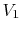with the rest of the insulated enclosure a vacuum. The total volume (plus the evacuated volume) is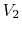. At a given time a hole is opened in the partition and the gas rushes through to fill the rest of the enclosure.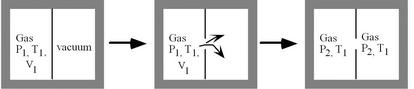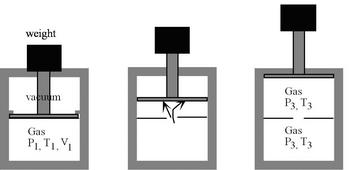During the expansion there is no work exchanged with the surroundings because there is no motion of the boundaries4.1. The enclosure is insulated so there is no heat exchange. The first law tells us therefore that the internal energy is constant (). For an ideal gas, the internal energy is a function of temperature only so that the temperature of the gas before the free expansion and after the expansion has been completed is the same. Characterizing the before and after states:

• Before: State 1,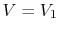,• After: State 2,,.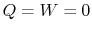, so there is no change in the surroundings.

To restore the original state, i.e., to go back to the original volume at the same temperature (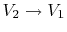at constant) we can compress the gas isothermally (using work from an external agency). We can do this in a quasi-equilibrium manner, with, as in Figure 4.5. If so the work that we need to do is. We have evaluated the work in a reversible isothermal expansion (Eq. 3.1), and we can apply the arguments to the case of a reversible isothermal compression. The work done on the system to go from state 2'' to state 1'' isFrom the first law, this amount of heat must also be rejected from the gas to the surroundings if the temperature of the gas is to remain constant. A schematic of the compression process, in terms of heat and work exchanged is shown in Figure 4.6.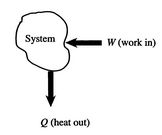At the end of the combined process (free expansion plus reversible compression):

1. The system has been returned to its initial state (no change in system state).
2. The surroundings (us!) did work on the system of magnitude4.2.
3. The surroundings received an amount of heat,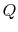, which is equal to.
4. The sum of all of these events is that we have converted an amount of work,, into an amount of heat,, withandnumerically equal in Joules.

The net effect is the same as if we let a weight fall and pull a block along a rough surface, as in Figure 4.7. There is 100% conversion of work into heat.The results of the free expansion can be contrasted against a process of isothermal expansion against a pressurewhich is slightly different than that of the system, as shown in Figure 4.8.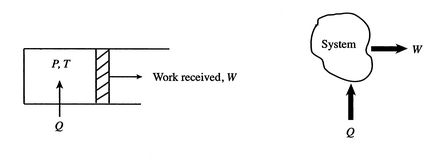During the expansion, work is done on the surroundings of magnitude, wherecan be taken as the system pressure. As evaluated in Eq. (3.1), the magnitude of the work done by the system is. At the end of the isothermal expansion, therefore:

1. The surroundings have received work.
2. The surroundings have given up heat,, numerically equal to.

We now wish to restore the system to its initial state, just as we did in the free expansion. To do this we need to do work on the system and extract heat from the system, just as in the free expansion. In fact, because we are doing a transition between the same states along the same path, the work and heat exchange are the same as those for the compression process examined just above.

The overall result when we have restored the system to the initial state, however, is quite different for the reversible expansion than for the free expansion. For the reversible expansion, the work we need to do on the system to compress it has the same magnitude as the work we received during the expansion process. Indeed, we could raise a weight during the expansion and then allow it to be lowered during the compression process. Similarly the heat put into the system by us (the surroundings) during the expansion process has the same magnitude as the heat received by us during the compression process. The result is that when the system has been restored back to its initial state, so have the surroundings. There is no trace of the overall process on either the system or the surroundings. That is another meaning of the word reversible.''

Muddy Points

With the isothermal reversible expansion is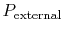constant? If so, how can we have? (MP 4.2)

Why is the work done equal to zero in the free expansion? (MP 4.3)

Is irreversibility defined by whether or not a mark is left on the outside environment? (MP 4.4)

UnifiedTP